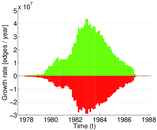# Yahoo songs

This is the bipartite person–song rating network used in the KDD Cup 2011. It contains ratings on a scale from 0 to 100 taken from Yahoo Music. This network contains over 250 million edges (i.e., ratings), and is one of the largest openly-available rating datasets.

 Code `YS` Internal name `yahoo-song` Name Yahoo songs Data source http://www.kdnuggets.com/2011/02/kdd-cup-2011-recommending-music.html AvailabilityDataset is available for download Consistency checkDataset passed all tests Category Rating network Node meaning Person, song Edge meaning Rating Network formatBipartite, undirected Edge type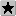Ratings, no multiple edges Temporal dataEdges are annotated with timestamps

## Statistics

 Size n = 1,625,951 Left size n1 = 1,000,990 Right size n2 = 624,961 Volume m = 256,804,235 Wedge count s = 4,627,224,528,654 Maximum degree dmax = 468,366 Maximum left degree d1max = 307,205 Maximum right degree d2max = 468,366 Average degree d = 315.882 Average left degree d1 = 256.550 Average right degree d2 = 410.912 Fill p = 0.000 410 506 Size of LCC N = 1,625,951 50-Percentile effective diameter δ0.5 = 2.236 31 90-Percentile effective diameter δ0.9 = 3.350 05 Mean distance δm = 2.760 80 Gini coefficient G = 0.752 830 Balanced inequality ratio P = 0.201 029 Left balanced inequality ratio P1 = 0.192 464 Right balanced inequality ratio P2 = 0.163 685 Relative edge distribution entropy Her = 0.871 153 Power law exponent γ = 1.403 07 Degree assortativity ρ = −0.103 038 Degree assortativity p-value pρ = 0.000 00 Spectral norm α = 130,625 Negativity ζ = 0.541 386

## Plots

### Degree distribution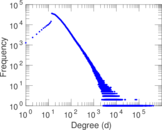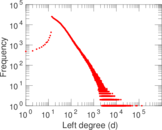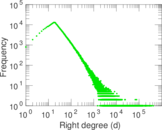### Cumulative degree distribution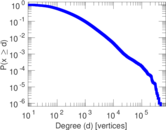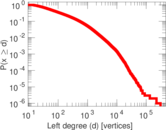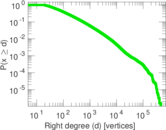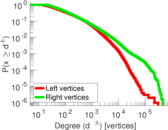### Lorenz curve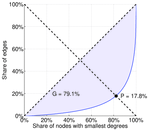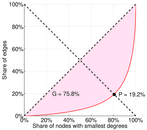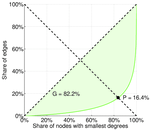### Spectral distribution of the adjacency matrix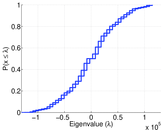### Spectral distribution of the normalized adjacency matrix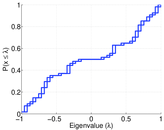### Spectral distribution of the Laplacian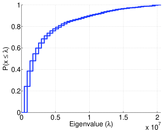### Spectral graph drawing based on the adjacency matrix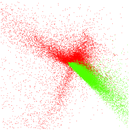### Spectral graph drawing based on the normalized adjacency matrix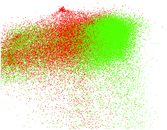### Degree assortativity### Hop distribution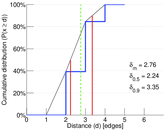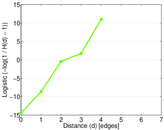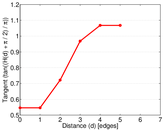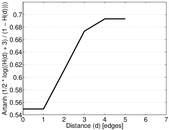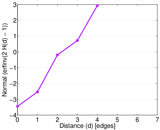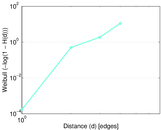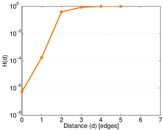### Edge weight/multiplicity distribution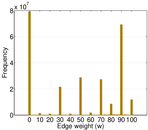### Temporal distribution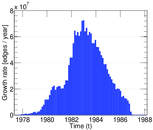### Diameter/density evolution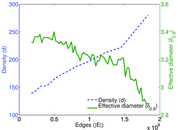### Signed temporal distribution